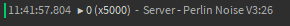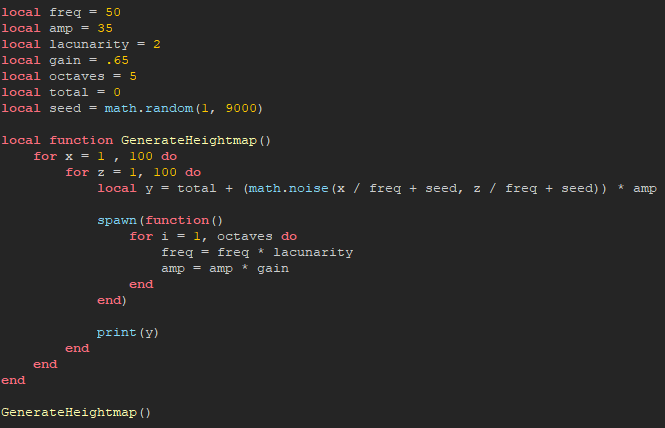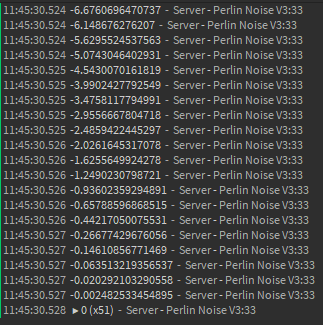# My function always returns 0

You can write your topic however you want, but you need to answer these questions:

1. What do you want to achieve? Keep it simple and clear!
I want my function to return numbers that aren’t only 0

2. What is the issue? Include screenshots / videos if possible!
My function only prints 0, but when I use a different method, it doesn’t only print 0.

The function that is meant to return numbers that aren’t only 0 but instead only returns 0

OutputThe function that uses the same math but uses a different method instead of being a function that returns the valueOutput1. What solutions have you tried so far? Did you look for solutions on the Developer Hub?
I have seen similar problems although they’re dealing with physical parts and are dealing with arrays.

Here are the scripts if you want to try them out for yourself.

Function that only returns 0

``````local freq = 50
local amp = 35
local lacunarity = 2
local gain = .65
local octaves = 5
local total = 0
local seed = math.random(1, 9000)

local function fBm(x, z)
local y = 0

for i = 1, octaves do
y = total + (math.noise(x / freq + seed, z / freq + seed)) * amp
freq = freq * lacunarity
amp = amp * gain
end

return y
end

local function GenerateHeightmap()
for x = 1 , 100 do
for z = 1, 100 do
local y = fBm(x , z)

print(y)
end
end
end

GenerateHeightmap()
``````

Function that doesn’t return 0

``````local freq = 50
local amp = 35
local lacunarity = 2
local gain = .65
local octaves = 5
local total = 0
local seed = math.random(1, 9000)

local function GenerateHeightmap()
for x = 1 , 100 do
for z = 1, 100 do
local y = total + (math.noise(x / freq + seed, z / freq + seed)) * amp

spawn(function()
for i = 1, octaves do
freq = freq * lacunarity
amp = amp * gain
end
end)

print(y)
end
end
end

GenerateHeightmap()
``````

If my question isn’t clear enough for you, just ask me and i’ll try to explain it as clear as I can.
Any help would be appreciated.

I think it is because the “i = 1, octave” for loop wasn’t in a function called by spawn() and y was being calculated inside of the loop. I’m not sure why, but you should look into Threading Code and Beginners Guide to Coroutines It will propbably give you a better understanding of the code. The code below works.

``````local freq = 50
local amp = 35
local lacunarity = 2
local gain = .65
local octaves = 5
local total = 0
local seed = math.random(1, 9000)

function fBm(x, z)
local y = total + (math.noise(x / freq + seed, z / freq + seed)) * amp

spawn(function()
for i = 1, octaves do
freq = freq * lacunarity
amp = amp * gain
end
end)

return y
end

local function GenerateHeightmap()
for x = 1 , 100 do
for z = 1, 100 do
local y = fBm(x,z)

print(y)
end
end
end

GenerateHeightmap()``````